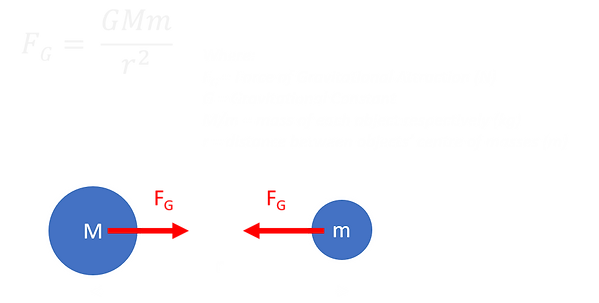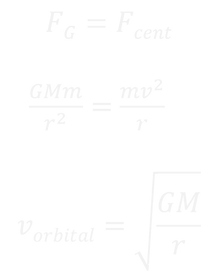top of page# 6.2. Newton's Law of Gravitation

Newton did a lot of good work - his three laws describing forces and motion, his work on light, inventing the branch of mathematics known as calculus - however, he is almost certainly best known for his theories surrounding gravity, with the famous story about the apple falling on his head.

Whether or not the apple on the head story is true, the apple tree certainly exists. One of the descendants of his original apple tree can still be found outside Trinity College Cambridge (typically surrounded by many tour groups).Newton's theory of gravity was (and remains) a very powerful tool, and gave a good mathematical description of the motion of the celestial bodies. It was finally superseded by subsequent theories of gravity (Einstein's general relativity), though it is applicable for the vast majority of applications (including all those you need to be worried about for the IB!). If you are interested in learning more about Einstein's theory of gravity, check out this video by Veritasium below.

Here, we're going to exclusively focus on Newtonian gravity. I've split the chapter up as follows:

• Newton's Law of Gravitation - A new equation for you, and the inverse square relationship

• Gravitational Field Strength - What does the fact that g = 9.81 Nkgˉ¹ actually mean?

• How can you be 'weightless' when gravity acts over an infinite range?

• Bringing in the previous work on circular motion to look at stuff in orbit

Newton's Law of Gravitation

#### Newton's Law of Gravitation

Gravity is one of the universe's four fundamental forces, alongside electromagnetism and the strong and weak nuclear forces. It is by far the weakest of the four forces, so is most noticeable when we are considering objects with very large masses (e.g. celestial bodies such as planets and stars). However, it does act between all massive objects.

Gravity has the following properties:

• It acts between all objects with mass

• It is always attractive

• It acts over an infinite range

Newton came up with a mathematical description of these ideas. TedEd have a nice video that introduces some of the key ideas introduced by Newton.

Newton's law of gravitation is a mathematical description of the size of the force of gravity between two objects with mass, separated by a distance 'r'.

(Note: By Newton's third law, each mass exerts an equal and opposite force on each other)The Gravitational Constant is a universal constant equal to 6.67 x 10ˉ¹¹ m³ kgˉ¹ sˉ². This constant of proportionality was originally determined through the Cavendish Torsion experiment (below).

Newton's Law of Gravitation is another example of an inverse square relationship, similar to Coulomb's Law previously explored in Section 5.1, i.e. that the Gravitational Force is proportional to the inverse of the square of the separation distance (F ∝ 1/r²). Isaac Physics have a nice lesson going through the Physics and geometry behind the inverse square law.

You can test this relationship using this PHET simulation. Change the size of the masses and the distance between them to see how the gravitational force changes.

(Note the application of Newton's Third Law here, the gravitational force acting on each sphere is equal and opposite.)

For a bit of practice, have a go at estimating the size of the gravitational force of attraction between:

i) The Earth and the Moon

ii) You and the Earth

iii) You and a book sitting on your desk

## The Cavendish Torsion Experiment

This relationship was experimentally tested in the lab by Henry Cavendish with the setup below.

Test the Cavendish experiment using this simulation from Geogebra. As you drag the slider to move the large lead spheres, the gravitational attraction induces torsion in the wire. By measuring the rotation of the spheres, Cavendish was able to experimentally measure the Gravitational Constant.

## Worked Example - Ratio of strength of electromagnetic and gravitational forces

Q. How many times stronger is the electrostatic force of attraction between a proton and an electron in a Hydrogen atom than the gravitational attraction?

For this question you will need the following information (all in your formula book!):

mass of an electron, me = 9.11 x 10ˉ³¹ kg

mass of a proton, mp = 1.67 x 10ˉ²⁷

charge on a proton/ electron, q = ±1.6 x 10ˉ¹⁹ C

Coulomb constant, k = 8.99×10⁹ kg m³ sˉ² Cˉ²

Gravitational constant, G = 6.67 x 10ˉ¹¹ m³ kgˉ¹ sˉ²

radius of a Hydrogen atom ≈ 1 x 10ˉ¹⁵ m (not actually needed!)We can now calculate the ratio of the two forces. Clearly at this scale the electromagnetic force is a great eal stronger than gravity. It is only on much larger mass scales (where charges are usually balanced) that the force of gravity is more significant.## Video Lessons

 Chris Doner Universal Gravitation and Satellites IB Specific Gradepod Gravitational Force IB Specific Khan Academy Introduction to Gravity Normal Force in an Elevator Study Nova Gravitation and Field Strength

## Resources

 IB Physics Topic 6 Notes IB-Physics.net Chapter 6 Summary IB Revision Notes Isaac Physics Inverse Square Laws Maths Gravitational Fields Mr. G 6.2 Teaching Notes 6.2 Student Notes

## Questions

 Cambridge University Press Topic 6: MCQs CUP Website Link Freely available online Grade Gorilla 6.2 (Gravitation) MCQ Topic 6 (Gravity/ Rotation) End Quiz Quick IB Specific Mixed MCQs Isaac Physics Newtonian Gravity Gravity and Orbits Mr. G 6.2 Formative Assessment Topic 6 Summary Qs IB Specific Questions Physics and Maths Tutor Gravitational Field Strength (AQA 2) Gravitational Field Strength MS (AQA 2) A-Level Qs: overlapping content
Gravitational Field Strength

#### Gravitational Field Strength

We have come across this idea of gravitational field strength when looking at the link between mass and weight. i.e. :

Weight (N) = Mass (kg) x gravitational field strength (N kgˉ¹)

We know that at the surface of Earth this value is equal to g = 9.81 N kgˉ¹.

(We remember that this also is the same as the initial acceleration due to gravity, 9.81 msˉ²)

Let's look more closely at this idea of field strength, and link it into our idea of Newtonian gravity. The gravitational field strength is defined as :

The gravitational force acting on a small unit mass (1 kg) at that point.

This is the same thing as the force per unit mass, and has units of Newtons per kilogram.

Formalising this as an equation, this becomes:

(Note, this is exactly the same as our W = mg equation above)If we divide through our equation for gravitational force by mass, we obtain a new equation for gravitational field strength. Note that although these equations look similar, the field strength is the force PER UNIT MASS at a certain point in space - this is therefore a useful property to describe a point within a gravitational field (e.g. the value for 'g' is the same for both a pencil and a person on the surface of the Earth).## Video Lessons

 Chris Doner Universal Gravitation and Satellites Gravity and Circular Motion IB Specific Chris Doner Universal Gravitation and Satellites Cowen Physics Gravitational Field Strength Khan Academy Centripetal Force and Gravitation Science Shorts Tension and Weight Study Nova Gravitation and Field Strength Orbital Motion

## Resources

 IB Physics Topic 6 Notes IB-Physics.net Chapter 6 Summary IB Revision Notes Mr. G 6.2 Teaching Notes 6.2 Student Notes

## Questions

 Cambridge University Press Topic 6: MCQs CUP Website Link Freely available online Grade Gorilla 6.2 (Gravitation) MCQ Topic 6 (Gravity/ Rotation) End Quiz Quick IB Specific Mixed MCQs Mr. G 6.2 Formative Assessment Topic 6 Summary Qs IB Specific Questions Physics and Maths Tutor Gravity & Orbits (Edexcel 1) Gravity & Orbits MS (Edexcel 1) Orbits (AQA 2) Orbits MS (AQA 2) A-Level Qs: overlapping content
Weight and Weightlessness

#### Weight and Weightlessness

What does it mean to be weightless? Does it mean that the force of gravity acting on you is zero? Well, we have seen through Newton's gravitational equation that gravity has an infinite range, so technically there is nowhere in the universe where the gravitational force acting on you is zero. There are plenty of videos on the ISS of astronauts experiencing 'weightlessness', so what is going on here, when they are still clearly under the influence of Earth's gravity. TedEd have a nice little video describing this phenomenon.

One of the best ways to think about this idea of 'weightlessness' is to consider the forces acting on your when in a lift. Imagine you are standing in a lift, standing on an ordinary set of weighing scales. Thinking about Newton's Third Law, the reading given by the weighing scales is actually a measure of the NORMAL REACTION force between you and the scales (equal and opposite to your reaction force up). Let's look at the three scenarios in the diagram below:

1) When travelling at a constant speed (up, down or stationary, it doesn't matter):

The forces on you are balanced (by Newton's First Law). This means that the reaction force reading on the weighing scales is equal to your weight (as you expect when you stand on your ordinary bathroom scales)

2) When you begin accelerating downwards (either moving up and slowing down, or moving down and speeding up):

As there is now a downwards acceleration, there must be a resultant force downwards. As your weight is fixed, this must mean that the reaction force decreases. The weighing scale reading would decrease, and you would feel less pressure on your feet.

If you know the acceleration, you could work out the magnitude of the resultant force using F=ma, then use this to work out the new reading on the scale.

3) If the lift cable snaps and you accelerate downwards at 9.81 msˉ²:

The resultant force is now equal to the weight force only. This means that the reaction force (and therefore reading on your weighing scales) would equal zero. You feel a sensation of 'weightlessness', as experienced by the astronauts on the ISS (for a short time at least until you reach the bottom of the lift shaft and experience a very rapid deceleration).This phenomenon is exactly what is experienced on the zero-gravity plane trips you may have seen (a.k.a. 'the Vomit Comet'). My fave Tom Scott does a nice little explanation of how these planes allow you to experience weightlessness whilst being very much under the influence of Earth's gravity.

Orbital Motion

#### Orbital Motion

We can combine the work we looked at in the previous section on Circular Motion with this section looking at Newton's Law of Gravitation to look specifically at objects in orbit. The orbital velocity is the linear velocity of a body in orbit.

PHET have a nice simulation here which allows you to look at the motion of planets in orbit. Here you can see that there is always a resultant force acting ont he orbiting planet, and that it is always directed towards the centre.

This means that the gravitational force is equal to the centripetal force.

Let's start by equating Newton's Law of Gravitation and our centripetal force equations. We can then rearrange for v to find our orbital velocity.## Worked Example - Find the height of a geostationary satellite

Geostationary satellites are positioned such that they have the same orbital period as the Earth (once every 24 hours). This means that they maintain a constant position over the same point on Earth, making them useful for communications.

Q. Calculate the height of a geostationary satellite above the surface of the Earth.

For this question you will need the following information:

mass of Earth mE = 6 x 10²⁴ kg

Gravitational constant, G = 6.67 x 10ˉ¹¹ m³ kgˉ¹ sˉ²

radius of Earth = 6400 km

We start by using our equations for orbital velocity. We know that the time period is equal to 24 hours, therefore we need to use our link between angular and linear velocity to introduce ω.